# XH110G

The maximum size of a card that fits in this model is 205 mm (L) × 120 mm (H) × 45 mm (D).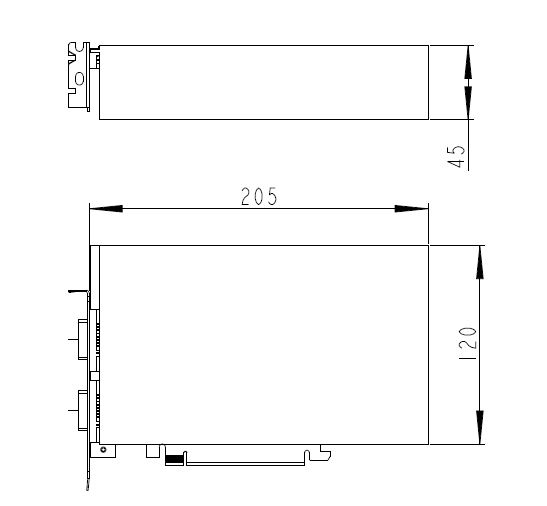The maximum permitted size is 11.5 x 28 x 88 mm.

The maximum permitted size for PCI-Express cards is 208.5 x 120 x 30 mm (LWH).

1. Unscrew the two thumbscrews of the chassis cover.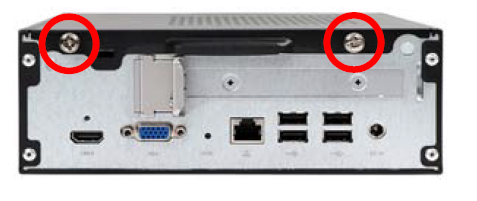2. Slide the cover backwards and upwards.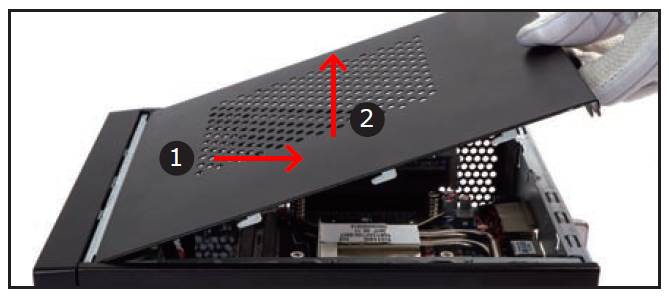1. Unfasten the four ICE module attachment screws.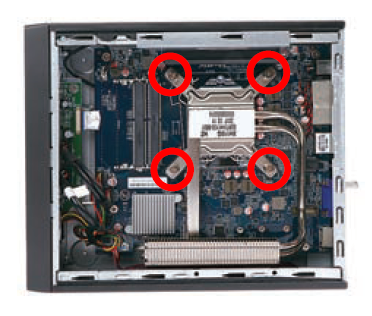2. Take the ICE module out of the chassis and put the module aside.

This 1151-pin socket is fragile and easily damaged. Always use extreme care when installing a CPU and limit the number of times you remove or change the CPU. Before installing the CPU, make sure to turn off the computer and unplug the power cord from the power outlet to prevent damage to the CPU.

→ Follow the steps below to correctly install the CPU in the motherboard CPU socket.

3. First unlock and raise the socket lever.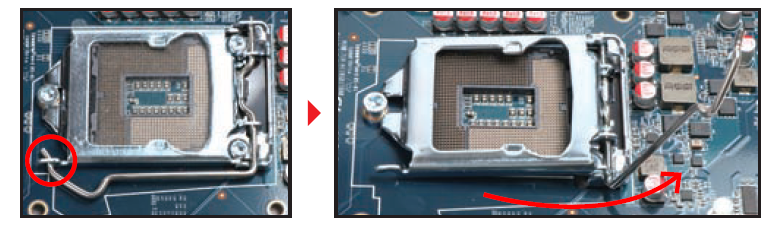4. Tear off the protective membrane from the metal load plate. Lift the metal load plate off the CPU socket.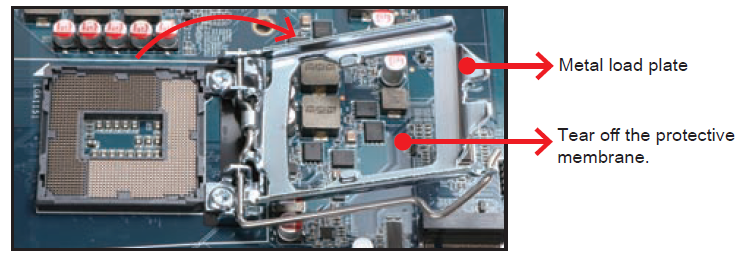DO NOT touch the socket contacts. To protect the CPU socket, always replace the protective socket cover when a CPU is not installed.

5. Orientate the CPU properly by aligning the CPU notches with the socket alignment keys. Make sure the CPU sits perfectly horizontal, then place the CPU on its socket.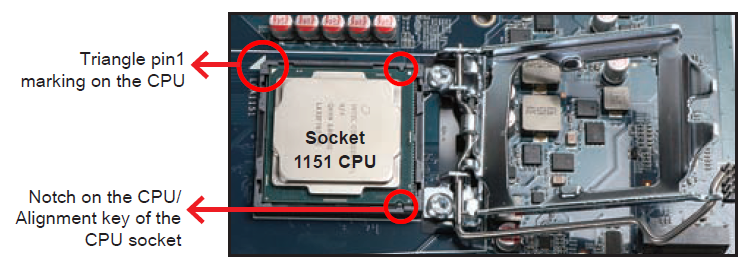Please be aware of the CPU orientation, DO NOT force the CPU on to its socket to avoid bending of pins hence damage of the CPU!

6. Close the metal load plate, lower the CPU socket lever and lock in place.
7. Spread thermal paste evenly on the CPU surface.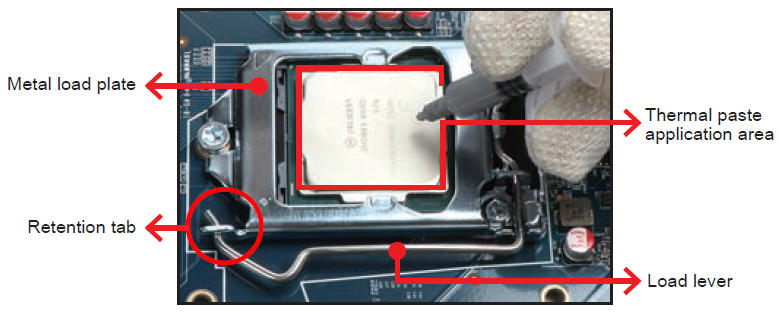Please do not apply excess amount of thermal paste.

8. Please look at the illustration below.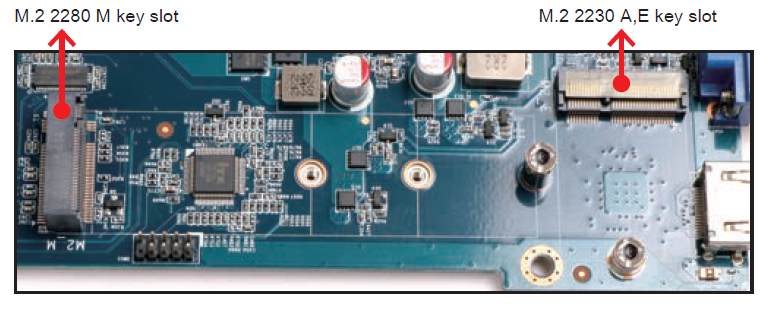9. Install the M.2 card into the M.2 slot and secure with the screw.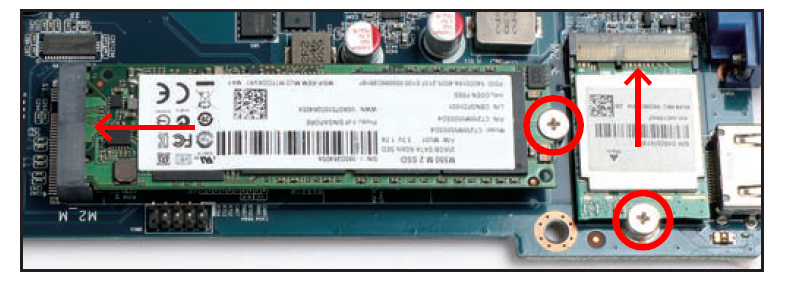10. Screw the ICE module on to the mainboard.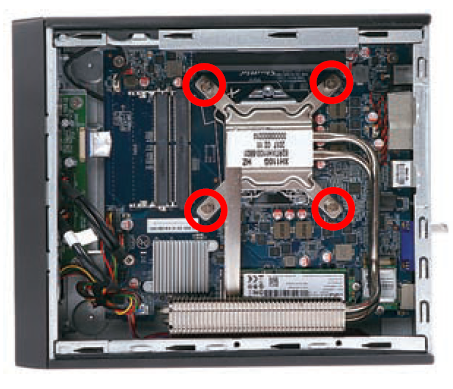This motherboard does only support 1.2 V DDR4 SO-DIMM memory modules.

1. Locate the two SO-DIMM slots on the mainboard.
2. Align the notch of the memory module with the one of the relevant memory slot.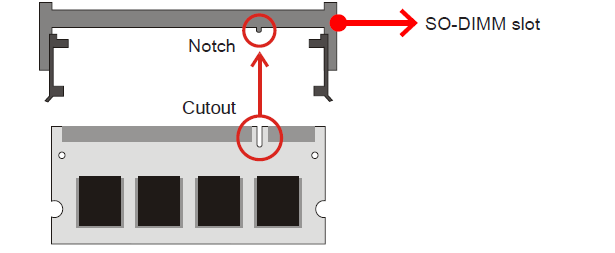3. Gently insert the module into the slot in a 45-degree angle.
4. Carefully push down the memory module until it snaps into the locking mechanism.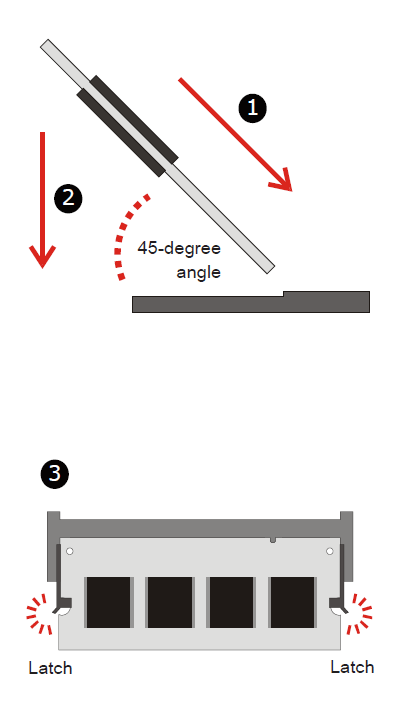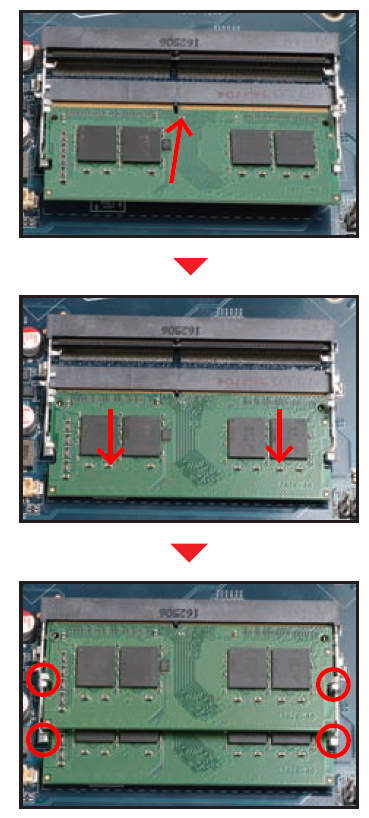5. Repeat the above steps to install an additional memory module, if desired.
1. Unfasten the screws of the expansion slot bracket. Remove the bracket and put it aside.

The maximum permitted size for display cards is 208.5 mm x 120 mm x 30 mm.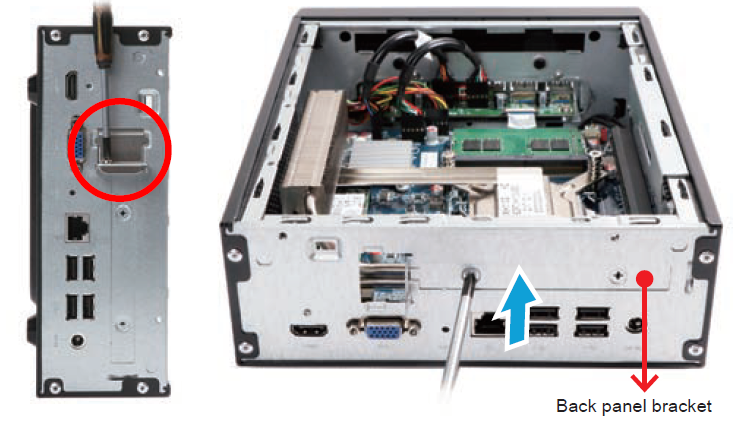2. Install the PCIe x16 card into the PCIe x16 slot.
3. Secure the graphics card by tightening one screw.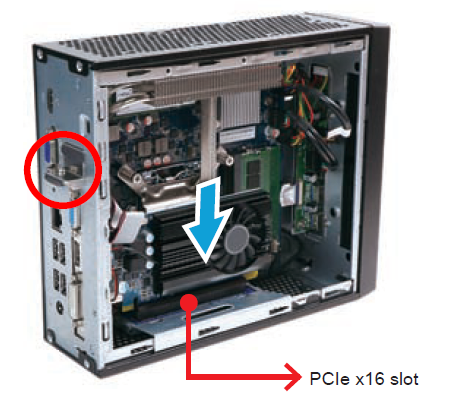1. Turn your XH110G upside down, then unscrew the screw of the HDD/SSD cover and remove it.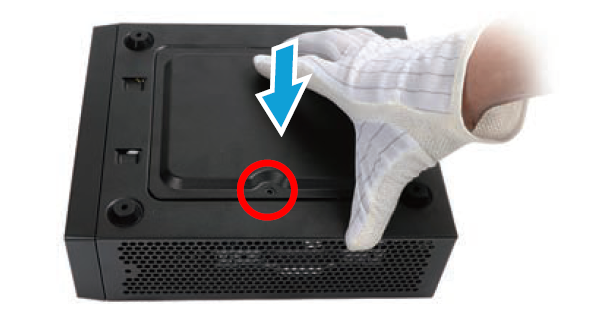2. Mount the HDD/SSD on the bracket with four screws.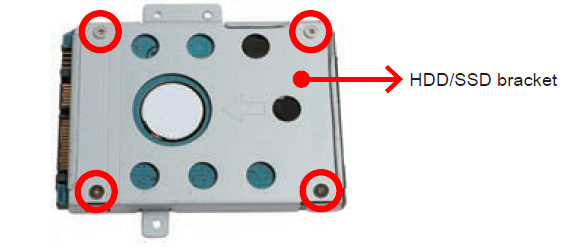3. Tear off the adhesive tape of the HDD/SSD cable. Install the HDD or SSD in the chassis using its bracket. Affix with three screws and connect the HDD or SSD with the afore mentioned cable. Insert a USB drive, if desired.

The maximum permitted size of an internal USB device is 11.5 mm x 28 mm x 88 mm.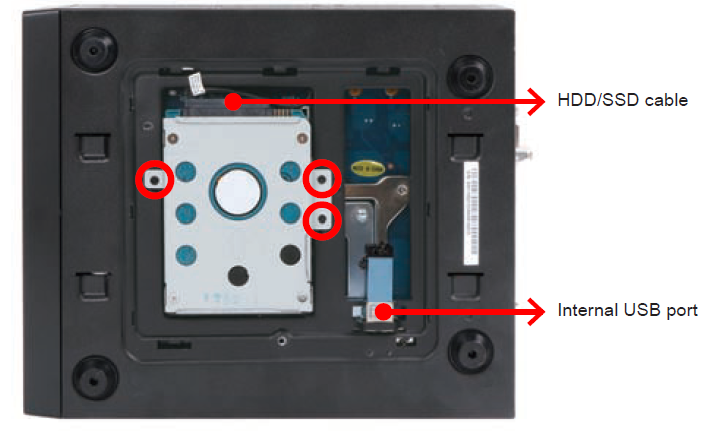4. Replace the HDD/SSD cover and also tighten the screw. Now turn your XH110G upside down again.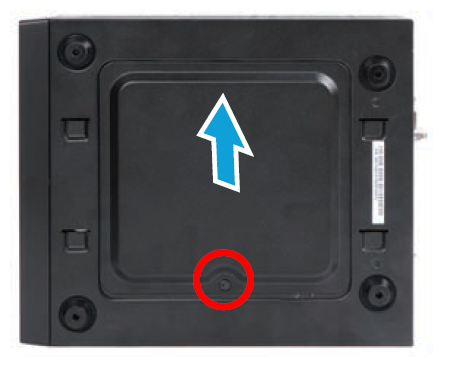5. Replace the cover and refasten the two thumbscrews.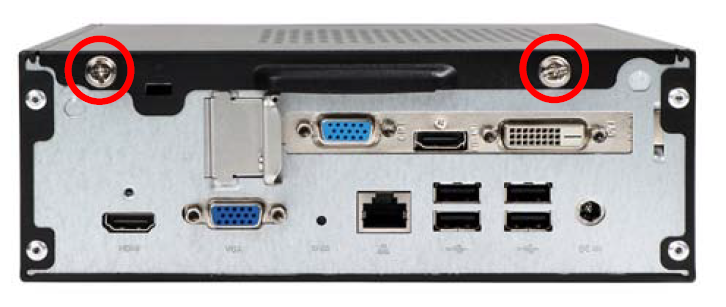6. Complete.

Please press the “Del” key while booting to enter BIOS. Here, please load the optimised BIOS settings.

The XH110G supports the 75×75 mm and 100×100 mm VESA standard. Installation happens as per illustrations below.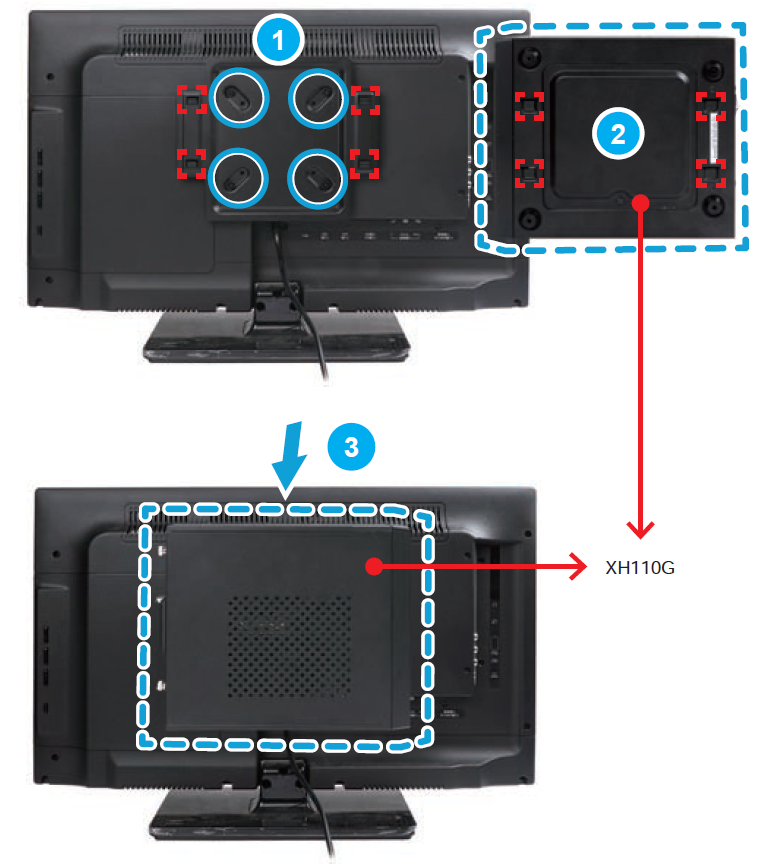```1=BATPWR
2=GND```
```1=OP(+)
2=GND```
```1=GND
2=USB2_D+
3=USB2_D-
4=+5VS```
```1=SATA_LED_+3.3V
2=SYS_LED_+3.3V
3=SATA_LED
4=GND
5=RST_nBTN
6=PWRSW-
7=GND
8=GND
9=N/C
10=N/A```
```1=CLK_DBG_24M
3=SIORST-
5=LFRAME-
6=+3.3V
8=GND
10=N/A```
```1=GND
2=+12V
3=FAN_TAC
4=FAN_CTL```
```1=MIC_L
2=GND
3=MIC_R
4=FP_AUDIO-JD
5=HP_R
6=MIC-JD
7=SENSE_B
8=N/A
9=HP_L
10=HP-JD```
```1=+5VS
2=+5VS
3=USB2_D-
4=USB2_D-
5=USB2_D+
6=USB2_D+
7=GND
8=GND
9=N/A
10=GND```
```1=N/C
2=N/C
3=N/C
4=N/C
5=GND
6=GND
7=GND
8=+5V
9=+5V
10=+5V
11=+5V
12=GND
13=GND
14=GND
15=SATA_TX+
16=SATA_TX-
17=GND
18=SATA_RX-
19=SATA_RX+
20=GND```
```1=GND
2=GND
3=USB2_D+
4=USB2_D-
5=GND
6=GND
7=USB3_TX+
8=USB3_TX-
9=GND
10=GND
11=USB3_RX+
12=USB3_RX-
13=+5VS
14=+5VS
15=+5VS
16=+5VS
17=USB2_D+
18=USB2_D-
19=GND
20=GND
21=USB3_TX+
22=USB3_TX-
23=GND
24=GND
25=USB3_RX+
26=USB3_RX-
27=GND
28=GND
29=GND
30=GND```